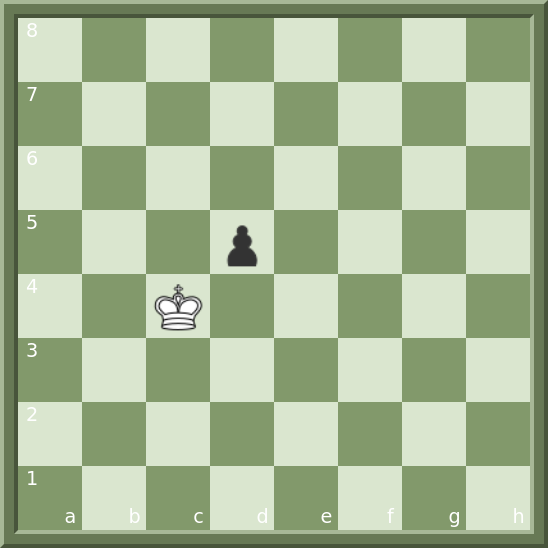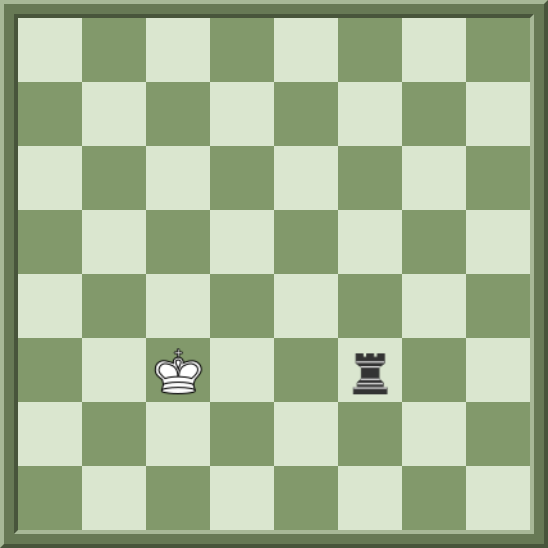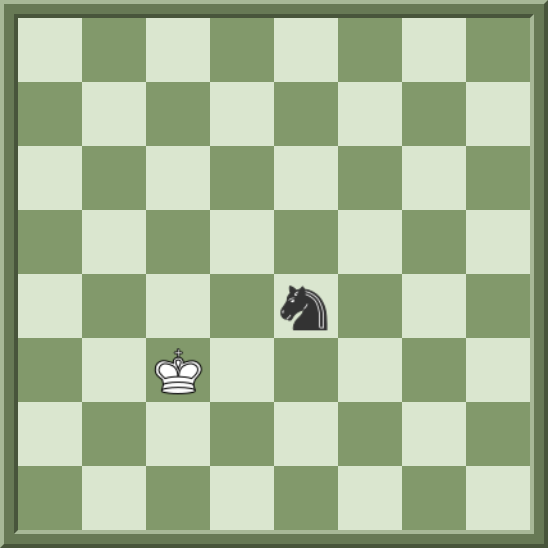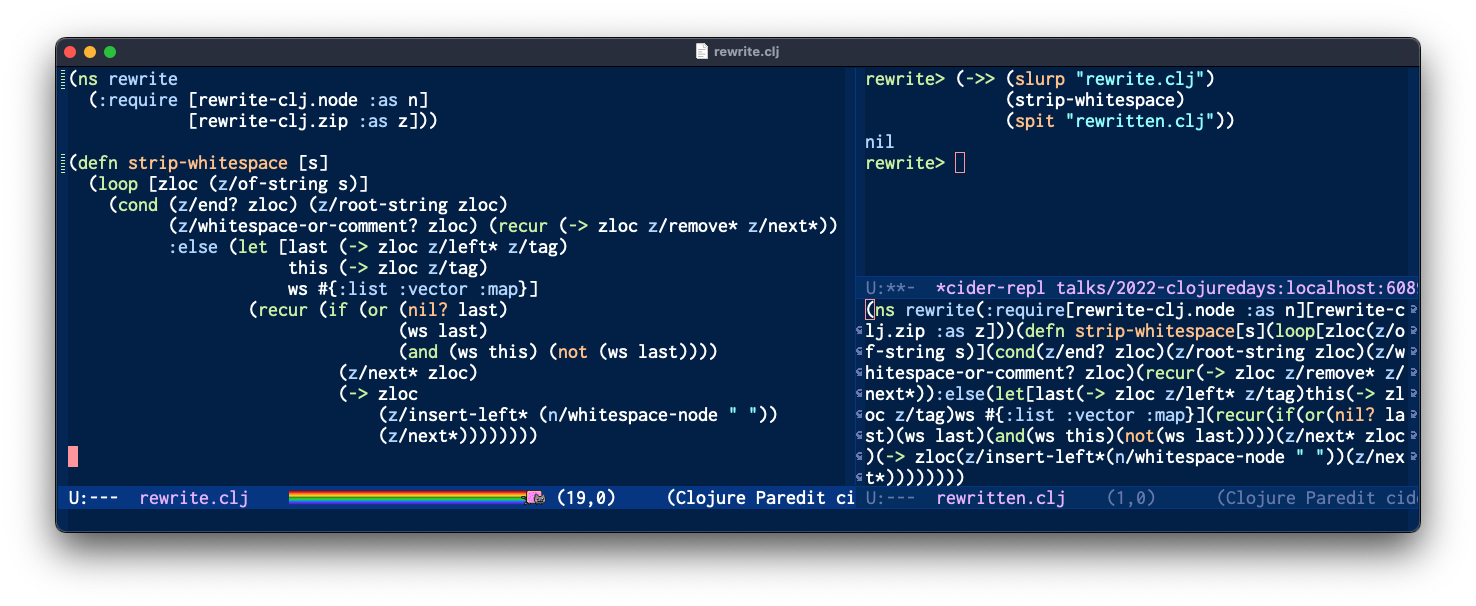# Golfing Clojure## Golfing Clojure

### Check checker in <280 characters of Clojure

#### Daniel Janus • ClojureDays • 2022-10-29## The Problem

You have to write a function `is-check` that takes for input a 8x8 chessboard in the form of a bi-dimensional array of strings and returns `true` if the black king is in check or `false` if it is not.

The array will include 64 squares which can contain the following characters: '♔', '♛', '♝', '♞', '♜', '♟', ' ' (a space).

There will always be exactly one king, which is the white king, whereas all the other pieces are black.

The board is oriented from White’s perspective.

``````
(deftest check-by-pawn
(is (true? (is-check
[[" "" "" "" "" "" "" "" "]
[" "" "" "" "" "" "" "" "]
[" "" "" "" "" "" "" "" "]
[" "" "" "" "" "" "" "" "]
[" "" "" ""♟"" "" "" "" "]
[" "" ""♔"" "" "" "" "" "]
[" "" "" "" "" "" "" "" "]
[" "" "" "" "" "" "" "" "]]
))))
``````## The Caveats

• The code must start with `(ns a)`
• The function must be called `is-check`
• The input must be a 8-vector of 8-vectors of 1-character strings
• Not a vector of vectors of characters
• Not a flat vector of strings
• The function must return `true` or `false`
• Not `nil`
• Not another truthy value
• The code must be at most 279 characters long, as measured by `String#length()`

## The Meta-Algorithm

``````
(def limit 279)

(defn too-long? [solution]
(> (count solution) limit))

(defn solve-golfed [problem]
(let [simple-solution (solve-simply problem)]
(->> (iterate chop-characters simple-solution)
(drop-while too-long?)
(first))))
``````

## The Simple Solution: Sketch

``````
(defn is-check [board]
(->> (fields board)
(filter has-black-piece?)
(filter checks-white-king?)
first
boolean))
``````

## Better Board Representation

``````

(defn board->map [board]
(->> board
(map-indexed
#(map-indexed
(fn [x y] [[x %] y])
%2))
(apply concat)
(into {})))

(defn is-check [board]
(->> board
board->map
(filter checks-white-king?)
first
boolean))
````````````
{[2 4] "♔",
[3 5] "♟",
[0 0] " ",
;; ...
}
``````

## Detecting Checks: Casts

``````
(defn coord+ [[x y] [dx dy]]
[(+ x dx) (+ y dy)])

(defn cast [board field delta]
(->> field
(iterate #(coord+ delta %))
next
(map board)
(remove #{" "})
first))

(defn rook-checks? [board field]
(-> (map (partial cast board field)
[[0 1] [0 -1] [1 0] [-1 0]])
set
(contains? "♔")))
````````````
(cast b
[5 5]
[-1 0])
;=> "♔"
``````

## Detecting Checks: Drops

``````
(defn drop-checks? [coords board field]
(-> (map #(board (coord+ field %))
coords)
set
(contains? "♔")))

(def knight-checks?
(partial drop-checks?
[[1 2]   [2 1]
[1 -2]  [2 -1]
[-1 -2] [-2 -1]
[-1 2]  [-2 1]]))

(def pawn-checks?
(partial drop-checks?
[[1 1] [-1 1]]))
``````## Putting it all together

``````
(def piece->checks
{"♝" bishop-checks?
"♜" rook-checks?
"♛" queen-checks?
"♞" knight-checks?
"♟" pawn-checks?})

(defn checks-white-king?
[board [field piece]]
(let [checks? (piece->checks piece (constantly false))]
(checks? board field)))
``````## Strip whitespace with rewrite-clj``````
(ns a)(defn board->map[board](->> board(map-indexed #(map-indexed(fn[x y][[x %]y])%2))(apply concat)(into{})))(defn coord+[[x y][dx dy]][(+ x dx)(+ y dy)])(defn cast[board field delta](->> field(iterate #(coord+ delta %))next(map board)(remove #{" "})first))(defn rook-checks?[board field](->(map(partial cast board field)[[0 1][0 -1][1 0][-1 0]])set(contains?"♔")))(defn bishop-checks?[board field](->(map(partial cast board field)[[1 1][1 -1][-1 -1][-1 1]])set(contains?"♔")))(defn queen-checks?[board field](->(map(partial cast board field)[[1 1][1 -1][-1 -1][-1 1][1 0][0 1][-1 0][0 -1]])set(contains?"♔")))(defn drop-checks?[coords board field](->(map #(board(coord+ field %))coords)set(contains?"♔")))(def knight-checks?(partial drop-checks?[[1 2][2 1][1 -2][2 -1][-1 -2][-2 -1][-1 2][-2 1]]))(def pawn-checks?(partial drop-checks?[[1 1][-1 1]]))(def piece->checks{"♝"bishop-checks?"♜"rook-checks?"♛"queen-checks?"♞"knight-checks?"♟"pawn-checks?})(defn checks-white-king?[board[field piece]](let[checks?(piece->checks piece(constantly false))](checks? board field)))(defn is-check[board](let[board(board->map board)](->> board(filter(partial checks-white-king? board))first boolean)))
``````

## Higher-order functions FTW

``````
(defn attack-checks? [move-fn]
(fn [deltas]
(fn [board field]
((set (map #(move-fn board field %) deltas)) "♔"))))

(def cast-checks?
(attack-checks? cast))

(def drop-checks?
(attack-checks? (fn [board field delta]
(board (coord+ field delta)))))
``````

## Shorter check checking

``````
(def rook-checks?
(cast-checks? [[0 1] [0 -1] [1 0] [-1 0]]))

(def bishop-checks?
(cast-checks? [[1 1] [1 -1] [-1 -1] [-1 1]]))

(def piece->checks
{"♝" bishop-checks?
"♜" rook-checks?
"♛" (some-fn bishop-checks? rook-checks?)
"♞" (drop-checks?
[[1 2]  [2 1] [1 -2] [2 -1]
[-1 -2] [-2 -1] [-1 2] [-2 1]])
"♟" (drop-checks? [[1 1] [-1 1]])})
``````

## All in one

``````
(defn is-check [board]
(let [board (into {} (apply concat (map-indexed #(map-indexed (fn [x y] [[x %] y]) %2) board)))
coord+ #(map + %1 %2)
cast (fn [x y] (first (remove #{" "} (map board (next (iterate #(coord+ x %) y))))))
attack-checks? (fn [g] (fn [P] (fn [f] ((set (map #(g % f) P)) "♔"))))
cast-checks? (attack-checks? cast)
drop-checks? (attack-checks? (comp board coord+))
rook-checks? (cast-checks? [[0 1] [0 -1] [1 0] [-1 0]])
bishop-checks? (cast-checks? [[1 1] [1 -1] [-1 -1] [-1 1]])
check-fns {"♝" bishop-checks?
"♜" rook-checks?
"♛" (some-fn bishop-checks? rook-checks?)
"♞" (drop-checks? [[1 2] [2 1] [1 -2] [2 -1] [-1 -2] [-2 -1] [-1 2] [-2 1]])
"♟" (drop-checks? [[1 1] [-1 1]])}]
(boolean (some boolean (for [[a f] board] ((check-fns f not) a))))))
``````

## Shorter identifiers

``````
(defn is-check [b]
(let [b (into {} (apply concat (map-indexed #(map-indexed
(fn [x y] [[x %] y]) %2) b)))
p #(map + %1 %2)
c (fn [x y] (first (remove #{" "} (map b (next (iterate #(p x %) y))))))
a (fn [g] (fn [P] (fn [f] ((set (map #(g % f) P)) "♔"))))
x (a c)
d (a (comp b p))
R (x [[0 1] [0 -1] [1 0] [-1 0]])
B (x [[1 1] [1 -1] [-1 -1] [-1 1]])
m {"♝" B
"♜" R
"♛" (some-fn B R)
"♞" (d [[1 2] [2 1] [1 -2] [2 -1] [-1 -2] [-2 -1] [-1 2] [-2 1]])
"♟" (d [[1 1] [-1 1]])}]
(boolean (some boolean (for [[a f] b] ((m f not) a))))))
``````

## Abbreviations

``````
(defn is-check [b]
(let [M map-indexed Z boolean
b (into {} (apply concat (M #(M (fn [x y] [[x %] y]) %2) b)))
p #(map + %1 %2)
a (fn [g] (fn [P] (fn [f] ((set (map #(g % f) P)) "♔"))))
x (a (fn [x y] (first (remove #{" "} (map b (next
(iterate #(p x %) y)))))))
d (a (comp b p))
R (x [[0 1] [0 -1] [1 0] [-1 0]])
B (x [[1 1] [1 -1] [-1 -1] [-1 1]])
m {"♝" B
"♜" R
"♛" (some-fn B R)
"♞" (d [[1 2] [2 1] [1 -2] [2 -1]
[-1 -2] [-2 -1] [-1 2] [-2 1]])
"♟" (d [[1 1] [-1 1]])}]
(Z (some Z (for [[a f] b] ((m f not) a))))))
``````

## Rotating deltas

``````
(defn is-check [b]
(let [M map-indexed Z boolean I iterate
b (into {} (apply concat (M #(M (fn [x y] [[x %] y]) %2) b)))
p #(map + %1 %2)
a (fn [g] (fn [P] (fn [f] ((set (map #(g % f) P)) "♔"))))
x (a (fn [x y] (first (remove #{" "} (map b (next
(I #(p x %) y)))))))
d (a (comp b p))
r #(take 4 (I (fn [[x y]] [(- y) x]) %&))
R (x (r 0 1))
B (x (r 1 1))
m {"♝" B "♜" R "♛" (some-fn B R)
"♞" (d (into (r 1 2) (r 2 1)))
"♟" (d [[1 1] [-1 1]])}]
(Z (some Z (for [[a f] b] ((m f not) a))))))
``````

## Going ASCII

``````
(defn is-check [b]
(let [M map-indexed Z boolean I iterate
b (into {} (apply concat (M #(M (fn [x y] [[x %] y]) %2) b)))
p #(map + %1 %2)
a (fn [g] (fn [P] (fn [f] ((set (map #(g % f) P)) "\u2654"))))
x (a (fn [x y] (first (remove #{" "} (map b (next
(I #(p x %) y)))))))
d (a (comp b p))
r #(take 4 (I (fn [[x y]] [(- y) x]) %&))
R (x (r 0 1))
B (x (r 1 1))
m {"\u265d" B "\u265c" R "\u265b" (some-fn B R)
"\u265e" (d (into (r 1 2) (r 2 1)))
"\u265f" (d [[1 1] [-1 1]])}]
(Z (some Z (for [[a f] b] ((m f not) a))))))
``````

## Change representation, again

``````
(defn is-check [b]
(let [M map-indexed Z boolean I iterate
b (into {} (apply concat (M #(M (fn [x y]
[[x %] (mod (int (first y)) 14)]) %2) b)))
p #(map + %1 %2)
a (fn [g] (fn [P] (fn [f] ((set (map #(g % f) P)) 12))))
x (a (fn [x y] (first (remove #{4} (map b (next
(I #(p x %) y)))))))
d (a (comp b p))
r #(take 4 (I (fn [[x y]] [(- y) x]) %&))
R (x (r 0 1))
B (x (r 1 1))
m {7 B 6 R 5 (some-fn B R)
8 (d (into (r 1 2) (r 2 1)))
9 (d [[1 1] [-1 1]])}]
(Z (some Z (for [[a f] b] ((m f not) a))))))
``````

## Cutting characters with a scalpel

``````
(defn is-check [b]
(let [M map-indexed Z boolean I iterate
b (into {} (apply concat (M #(M (fn [x y]
[[x %] (mod (int (first y)) 14)]) %2) b)))
p #(map + % %2)
a (fn [g] (fn [P] (fn [f] ((set (map #(g % f) P)) 12))))
x (a (fn [x y] (first (remove #{4} (map b (next
(I #(p x %) y)))))))
d (a (comp b p))
r #(take 4 (I (fn [[x y]] [(- y) x]) %&))
R (x (r 0 1))
B (x (r 1 1))
m {6 R 5 (some-fn B R)
8 (d (into (r 1 2) (r 2 1)))
9 (d [[1 1] [-1 1]]) 7 B}]
(Z (some Z (for [[a f] b] ((m f not) a))))))
``````

## Moar refactoring

``````
(defn is-check [b]
(let [q (range 8)
b (zipmap (for [x q y q] [y x])
(for [[x] (flatten b)]
(mod (int x) 14)))
p #(map + % %2)
a (fn [g] (fn [P] (fn [f] ((set (map #(g % f) P)) 12))))
x (a (fn [x y]
(loop [a (p x y)]
(if (= (b a) 4) (recur (p x a)) (b a)))))
d (a (comp b p))
r #(partition 2 1 [% %2 (- %) (- %2) %])
v (r -1 1)
R (x (r 0 1))]
(not-every? not
(for [[a f] b]
(({5 (some-fn (x v) R), 8 (d (into (r 1 2) (r 2 1))),
9 (d (take 2 v)), 7 (x v), 6 R} f not)
a)))))
``````

## Coup de grace

2 ASCII characters -> 1 UTF-16 code unit

``````
(defn compress [s]
(->> s
.getBytes
(partition-all 2)
(map (fn [[a b]] (+ (* 128 a) b)))
(map #(Character/toString %))
(apply str)))
``````
``````
(->> "COMPRESSED CODE"
(mapcat #((juxt / mod) (int %) 128))
byte-array
String.
eval)
``````
``````
(ns a)(->>"ᑤ㋦㜠㓳ᛣ㑥㇫ⷢ⺨㙥㩛㢨㥡㝧㊠ᰩㄨ㵩㡭ヰᑦ㟲ⷸၱၹၱ⻛㲠㱝ᒨ㍯㥛ⷸ⺨㍬ヴ㩥㜠ㄩ⺨㛯㈨㓮㨠㰩ᢴᒩᓰဣᑭヰါဥဥᤩエ㍮ⷧ⺨㍮ⷐ⺨㍮ⷦ⺨ᑳ㋴ᑭヰဣᑧဥၦᓐᒩᢲᒩᒩ㰨エ㍮ⷸၹ⺨㙯㟰ⷡᑰၸၹᓝᑩ㌨Ẩㄠォᨩᑲ㋣㫲ᑰၸၡᒩᑢၡᒩᒩᓤᑡᑣ㟭㠠ㄠ㠩ᓲဣᑰヲ㩩㩩㟮ဲေⶥဥᤨᚠኩᐭဥᤩዝᓶᑲိᢠᢩ⤨㰨㤠ᠠᢩᓝᑮ㟴ᛥ㭥㥹ᾠ㝯㨨㍯㥛ⷡၦ⻢⺨ᑻ᪨㧯㛥ᛦ㜨㰠㬩⤩ᰨ㈨㓮㩯ᑲေဲᒨ㤠ᤠᢩᒩᲨ㈨㩡㗥ဲၶᒩᮨ㰠㬩ᬠ⥽㌠㝯㨩ォᒩᒩ"(mapcat #((juxt / mod)(int %)128))byte-array String. read-string eval)
``````

## Another algorithm...

``````
(ns a)(defn is-check[b](let[r(range 8)z #(first(get-in b %))](=\♔(some #{\♔}(for[i r j r[p u]{\♟[[1 -1]]\♝[[1 -1]]\♜[[0 -1]]\♛[[0 1]]}s u :when(#{p}(z[i j]))](z(first(drop-while #(if(#{\♝\♜\♛}p)(=\space(z %)))(next(iterate(partial map + s)[i j]))))))))))
``````
— codewars.com/users/dxholmes